# Algebra

#### FUNCTIONS-1

Linear Equations: 1

Linear Equations:

Linear Equations is the easiest of all topics in Algebra and forms the starting point for the study of Algebra. Linear equation is an algebraic equation whose degree is one i.e. the highest exponent of the variable is one. Linear Equations may contain only one variable or more than one variable and is usually associated to solve word problems on the unknown quantities.  The graph of a linear equation is simply a straight line that can be depicted on the graph paper. In this lesson we shall try to understand the different types of linear equations based on the number of variables involved with focus on forming equations and solving them. At the end we shall see how to solve special equations on two variables that have only one equation which can have multiple solutions satisfying the equation.

Linear Equations on One Variable:

The general form a linear equation in one variable is ax+b =0 where a is the coefficient of the variable where a ≠ 0 and b is the constant term.

Solving such an equation, gives a unique value of the variable.

Example1 : Six years ago, the age of Tom and Jerry was in the ratio of 5:7 and 12 years from now their ages will be in the ratio of 4:5. What will be their ages 20 years from now?

Solution: Let the ages of Tom and Jerry six years ago were 5x and 7x

After 12 years, the age of Tom will be 5x + 6+12 = 5x + 18

Similarly after 12 years, the age of Jerry will be 7x+18

At this point their ages are in the ratio of 4:5, soSo, their present ages are 5x+6 and 7x +6 i.e. 36 and 48 years.

20 years from now, their ages will be 36+20 = 56 and 48+20 = 68 years respectively.

Example 2: A fruit vendor has some apples who which one third are rotten. He sells 4 not rotten apples while of the left two more apples become rotten. As a result of this the numbers of rotten apples become half of the total apples left. How many apples did he have earlier which were not rotten?

Solution: Let the total number of apples be x

Rotten Apples = 1/3 x

Not rotten = 2/3 x

Now he sells 4 un-rotten apples and also, 2 apples become rotten.

Un rotten apples = 2x/3 – 4 – 2 = 2x/3 -6

Number of rotten apples = x/3 + 2 ( As two un rotten apples become rotten)

Now, the number of rotten apples = Number of un rotten apples

x/3 + 2 = 2x/3 – 6

or x/3 = 8  or x = 24.

Initial number of un rotten apples = 2x/3 = (2/3)* 24 = 16

Example 3: Ramu worked at a company for which he was to be paid 48,000 plus a scooter for one year. He worked for eight months and received 28,000 and the scooter. What was the value of the scooter?

Solution: Let the value of the scooter be Rs x

He received scooter and 48,000 for one year. So monthly value = (48000+x)/12

He worked for 8 months, so the value he was to be given = 8(48000+x)/12

And he received Rs 28,000 and the scooter.

28000 + x = 8(48000+x)/12

3(28000+x) = 2(48000+x)

84000+3x = 96000 + 2x

Or x = 12,000

Thus, the worth of the scooter was Rs 12,000.

Example 4: A fraction has denominator one more than twice the denominator. If the numerator is increased by 1 while the denominator is decreased by 1, the new fraction becomes 2/3. What was the numerator of the original fraction?

Solution : Given that the denominator is one more than twice the numerator.

Let the numerator be ‘x’, so denominator will be 2x+1

Original fraction = x/(2x+1)

New fraction = (x+1)/(2x+1-1) = (x+1)/2x

According to the problem

x+12x=23

Or 3x+3 = 4x

X = 3

Thus, numerator of the original fraction is 3.

Example 5:  In the town Baarishpur, either it rained in the mornings and not in the evenings or it rained in the evenings but not in the mornings or it didn’t rain at all on either the mornings or evenings. In a month of 30 days the number of days when it rained in the morning were one more than the days when it rained in the evenings. Also, the total number of days it rained were 3 more than twice the number of days it didn’t rain. Then what is the number of evenings that didn’t experience rainfall in Baarishpur?

Solution: The language of the problem is a bit confusing, but if one breaks it into parts, the entire problem becomes easy to solve. Let the number of days in which it rained in the evenings but not in the mornings is x.

 Days Mornings Evenings X+1 Rained Didn’t rain X Didn’t rain Rained 30-(x+1+x)= 29-2x Didn’t rain Didn’t rain

Also, the total number of days it rained were 3 more than twice the number of days it didn’t rain.

Number of days it rained = x +x+1 = 2x+1

Number of days it didn’t rain = 29-2x

2x+1 =  3+ 2(29-2x)

2x + 1 = 61 -4x

6x = 60  or x = 10.

Number of evenings that didn’t experience rainfall = x+1 + 29-2x = 30 –x = 20

Linear Equations in Two or more Variables:

Two Variables: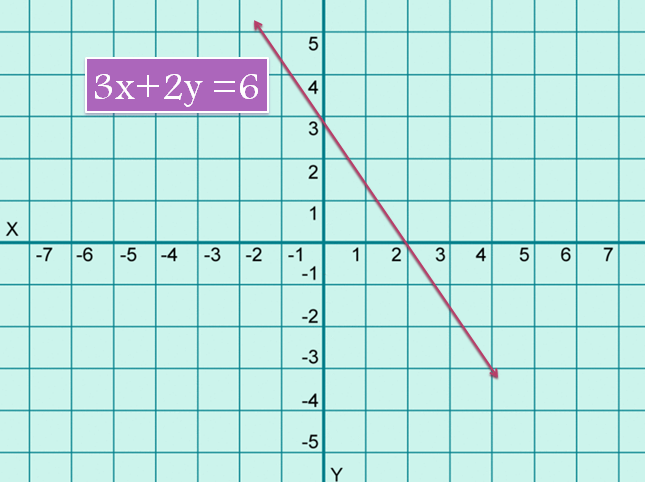A linear equation in two variables is of the form ax+by = c, where a and b are not equal to zero. The above equation represents a straight line with a slope of (–a/b).

Determining the conditions for Unique Solution, No solution and Infinite Solutions:

Condition for a Unique Solution: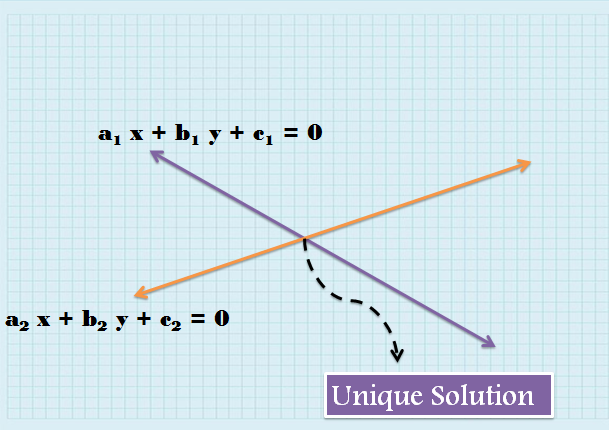Let’s say there are two linear equations a1x +b1y +c1 =0 and a2x + b2y +c2 =0. Now, these two equations represent two lines. For a unique solution to exist, these lines must intersect at a point.

Condition: a1/a2 ≠ b1/b2  i.e. their slopes must not be equal to each other.

Condition for No Solution: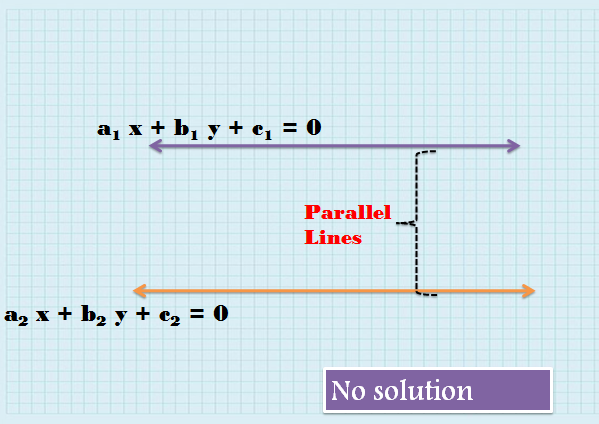If equations a1x +b1y +c1 =0 and a2x + b2y +c2 =0 have no solution, then that means that they lines are parallel to each other or their slopes must be equal but they must not be coincident lines.

Condition: a1/a2 = b1/b2 ≠ c1/c2 i.e. their slopes must not be equal to each other.

Condition for Infinite  Solution: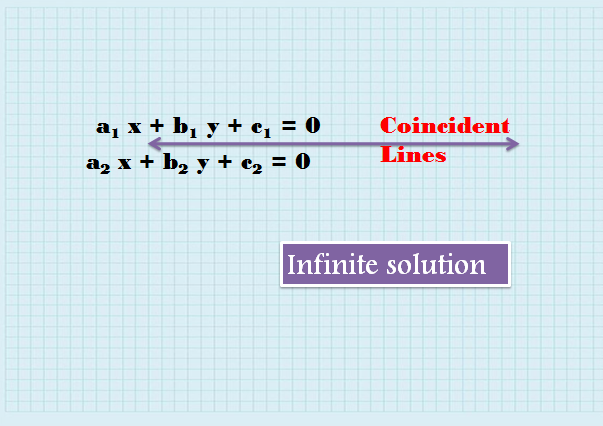If equations a1x +b1y +c1 =0 and a2x + b2y +c2 =0 have infinite solutions, then that means that the lines coincident.

Condition: a1/a2 = b1/b2 =c1/c2 i.e. their slopes must not be equal to each other.

Example 6: Find the value of k and p, such that the following system of equations have infinite solution?

(k-2)x – (p+1)y= 5

(2k-1)x -21y= 15

Solution:  For infinite solutions, the lines must be coincident or

a1/a2 = b1/b2 = c1/c2

k-22k-1=-(p+1)-21=515

k-22k-1=13   and    p+121=13

This further gives the values as k = 5 and p = 6.

Word problems on Two or More variables:

Example 7: Mehanti Lal is employed in a company on a contractof 30 days such that he will receive Rs 240 for the days he worked while 60 Rs per day will be fined for the number of days he remains absent. If at the end of 30 days he received Rs 1800, then how much fine did he pay?

Solution: Let the number of days he worked = x and the number of days he remained absent were y.

X+y = 30 (1) { As the contract lasts for 30 days}

Also, he received a total of Rs 1800.

240x – 60y = 1800 or 4x – y = 30  (2)

Solving (1) and (2) we get x = 12 and y = 18

So, total fine paid by Mehanti Lal = 18*60 = Rs 1080

Example 8: Arvind buys some pencils at Rs 4 per pencil and some pens at Rs 10 per pen. He makes 20% profit on selling the pencils and 10% profit on the pens. He received a total amount of Rs 3720. If the number of pencils were 20 more than four times the number of pens, then how many pencils did he buy?

Solution: Let the number of pencils and pens bought by Arvind were ‘x’ and ‘y’

x = 20 + 4y   (1)

Total cost of buying x pencils = 4x and the total cost of buying y pens = 10y Revenue earned by selling x pencils = 1.2*4x = 4.8x

Revenue earned by selling y pens = 1.1*10y = 11y

Given: 4.8x + 11y = 3720   (2)

4.8(20+4y) + 11y = 3720 { from (1)}

96 + 19.2y + 11y = 3720

30.2 y = 3624

Or y = 120 and x = 500

Thus, Arvind bought 500 pencils.

Example 9: There are two sections A and B. A student from section says, “ If six students from our section get transferred to section B, then there will twice as many students in section B as compared to our section. Then, a student from section B says. “If six students from our section gets transferred to section A, then there will be equal number of students in both the section. How many students were there in both the sections?

Solution: Let the number of students in section A and B be x and y respectively.

According to the first condition,

2(x- 6) = y+6   or 2x – y = 18  (1)

According to the second equation,

y-6 = x + 6

or y –x = 12    (2)

Solving (1) and (2) we get x = 30 and y = 42.

Example 10: Naruto is now a third as old as Kakashi was when Kakashi was a fourth as Naruto is now. If Kakashi is less than 18 years and all the ages are whole numbers, then what is the current age of Naruto?

Solution:  Let the present age of Kakshi is x and the past age of Kakashi was y years.

Accordingly :

 Naruto Kakashi Present y/3 x Past x/4 y

But as we talking about the same number of years back in the past, so the difference between the present and past ages of Naruto must be equal to the difference between the present and past ages of Kakashi

y/3 – x/4  = x – y

4y/3 = 5x/4

Or y = 15x/16

As the ages are integers, so x must be a multiple of 16 and as x is less than 18, so x =1 6

Thus, y = 15

# Or Naruto’s present age = y/3 = 15/3 = 5

Example 11: In a hostel, 4/9th of the girls watch Suits while 5/6th of the girls watch Game of Thrones. If 60girls watch both Suits and game of Thrones, then how many girls are there in the hostel if each girl watches atleast on TV series among the two?

Solution: Let the number of girls in the hostel = x

Number of girls watching Suits = 4x/9

Number of girls watching game of Thrones = 5x/6

Also 84 girls watch both Suits and game of Thrones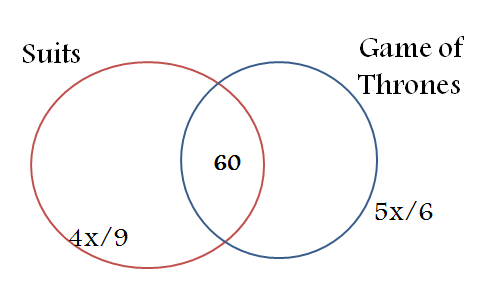x = 4x/9 + 5x/6 – 60

60 = 4x/9 + 5x/6 –x

60 = 5x/18

Or x = 216

Thus, there are 216 girls in the hostel.